# Excel Function: UNIQUE

The Excel function UNIQUE returns the list of unique values from a range of cells or a table.

Usage:

`=UNIQUE(array)`

or

`=UNIQUE(array, by_column, unique_values)`

## List of Unique Values

In this example, the aim is to obtain the unique list of countries from column A: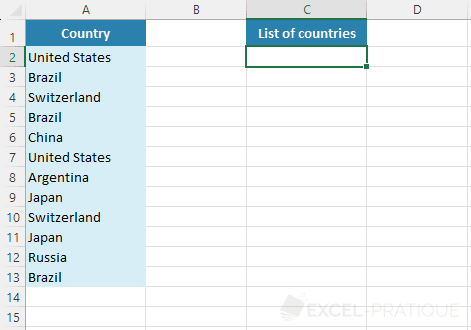Select the UNIQUE function and simply enter the cell range of the countries to obtain the unique list of countries:

``=UNIQUE(A2:A13)``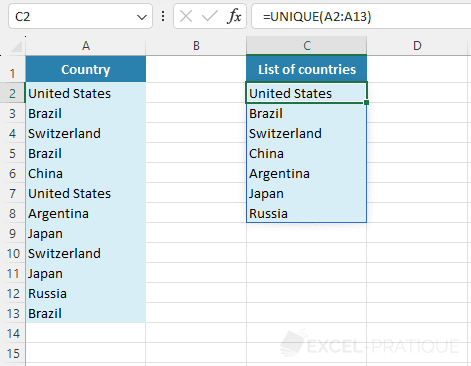To obtain a sorted list of countries, you can add the SORT function:

``=SORT(UNIQUE(A2:A13))``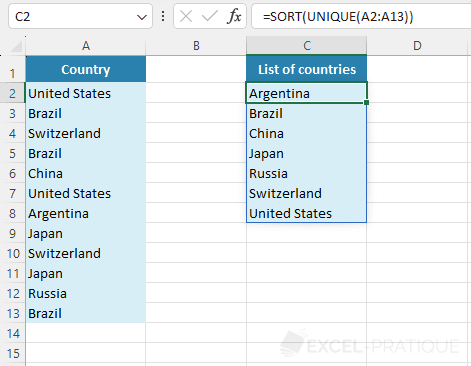If you select a range containing several columns, the UNIQUE function will return the list of unique rows:

``=UNIQUE(A2:B13)``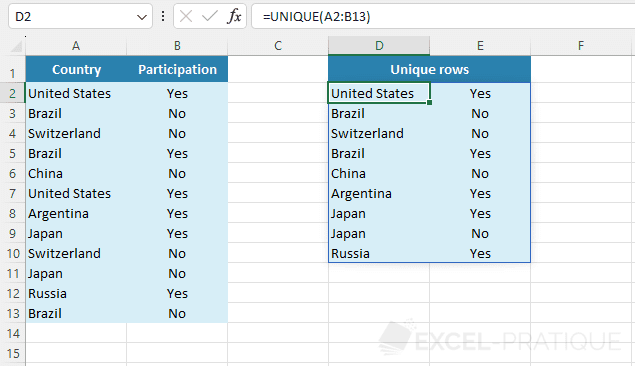## Search by Column

By default, the search for unique values is carried out row by row.

To list the unique columns, enter the value 1 (or TRUE) as the second argument:

``=UNIQUE(B1:M2,1)``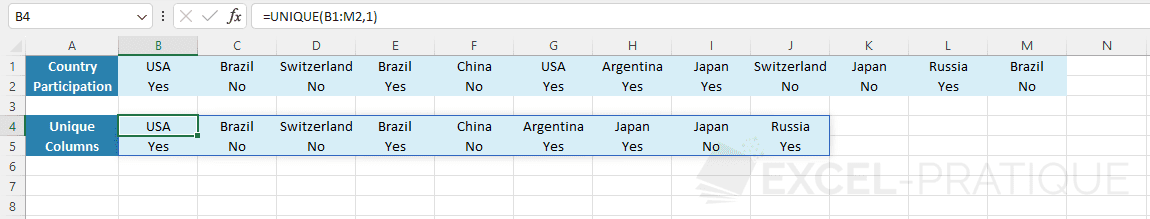## Search for Unique Values

To get only the values present once in the table, enter the value 1 (or TRUE) as the third argument:

``=UNIQUE(A2:B13,,1)``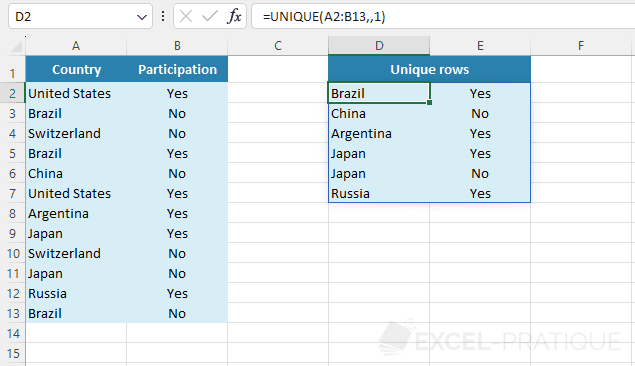For instance, the row Brazil No is present more than once in the table, so it is not listed here.